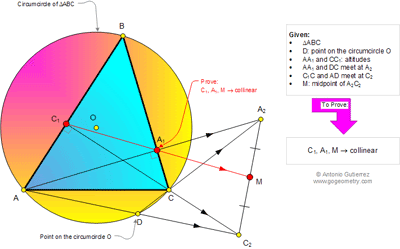## Sunday, February 16, 2014

### Math, Geometry Problem 984. Triangle, Circumcircle, Altitudes, Midpoint, Collinear Points

Geometry Problem
Level: Mathematics Education, High School, Honors Geometry, College.

Click the figure below to see the complete problem 984.#### 3 comments:

1.Let CC1 cut AA1 and circle O at H and E
Let C1A1 cut A2C2 at M
1. Note that C1E=C1H
Since ∠(A1HC)= ∠(CDC2)= ∠ (B) => quadrilateral HDC2A2 is cyclic
So ∠(HA2C)= ∠(CC2D)
Triangle HCA2 similar to triangle EAC2…. (case AA)
With corresponding altitudes CA1 and AC1

2. So A1H/A1A2=C1E/C1C2
Replace C1E=C1H in above => C1H/CC2=A1H/A1A2
or C1H/CC2 x A1A2/A1H=1 …(1)

3. Apply Menelaus’s theorem in triangle HA2C2 with secant C1A1M
C1H/C1C2 x MC2/MA2 x A1A2/A1H =1
Replace value of ( 1) to above expression we have MC2/MA2=1
So M is the midpoint of A2C2

2.See below for the sketch of problem 984
http://s25.postimg.org/b91r2quv3/pro_984_1.png
Peter Tran

3.Let A1C1 meet A2C2 at M'. Using Law of sine,
M'C2=sin(<CC1A1)*C1C2/sin(<C1M'C2)
and M'A2=sin(<A2A1M')*A1A2/sin(<A1M'A2)
Evaluate M'C2/M'A2=(sin(<CC1A1)*C1C2)/(sin(<A2A1M')*A1A2),
but sin(<CC1A1)/sin(<A2A1M')=A1C/AC1, which simplifies M'C2/M'A2 to
(A1C*C1C2)/(AC1*A1A2)=A1C/A1A2*C1C2/AC1=1 because triangles AC1C2 and CA1A2 are similar.
This proves M' to be midpoint of A2C2